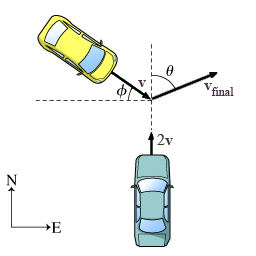###### FREE Expert Solution

x-axis points east, while the y-axis points north

(a)

v1x = 0 m/s

p1x = m1v1x = 0

v2x = vcosθ

p2x = m2v2x = mvcosϕ

83% (473 ratings)###### Problem Details

Two cars, both of mass m, collide and stick together. Prior to the collision, one car had been traveling north at speed 2v, while the second was traveling at speed v at an angle ϕ south of east. After the collision, the two-car system travels at speed vfinal at an angle θ east of north.(a) Find the initial momentum of the east-west components. Express your answer in terms of m, v, and ϕ

(b) Find the initial momentum of the north-south components. Express your answer in terms of m, v, and ϕ

(c) Find the final momentum of the east-west components. Express your answer in terms of m, vfinal and ϕm, vfinal and ϕ.

(d) Find the final momentum of the north-south components. Express your answer in terms of m, vfinal and m, vfinal and.

(e) Find the magnitude of the speed vfinal. Express your answer in terms of v, and ϕ.